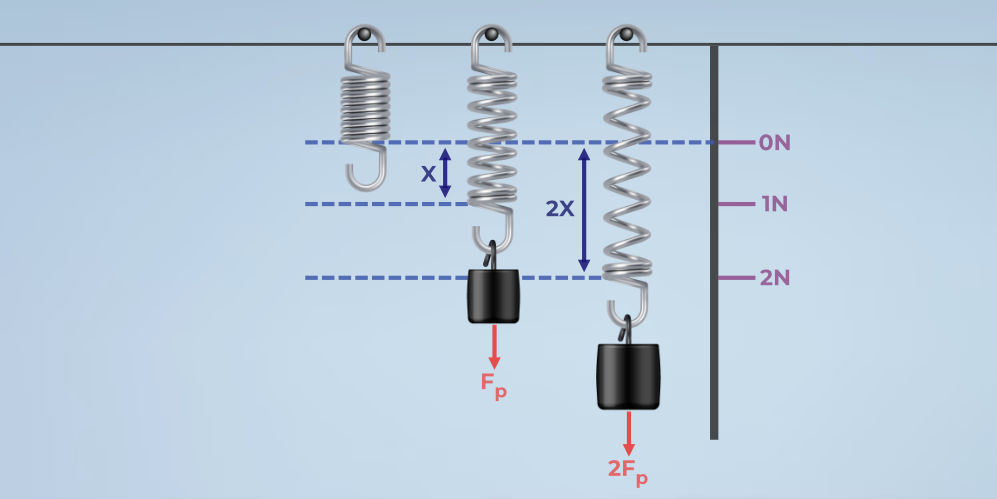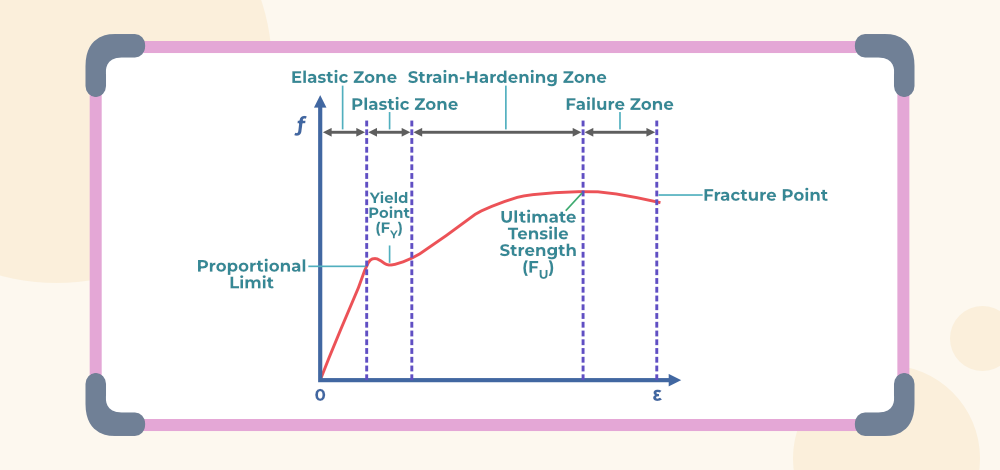GFG App
Open AppBrowser
Continue

Hooke’s law provides a relation between the stress applied to any material and the strain observed by the material. This law was proposed by English scientist Robert Hooke. Let’s learn about Hooke’s law, its application and other in detail in this article.

## What is Hooke’s Law?

According to Hooke’s law, the force required to extend or compress a spring by a certain distance is directly proportional to that distance. The spring stiffness is a constant factor feature. The property of elasticity indicates that stretching a spring twice as long requires twice as much power. Hooke’s law is the linear relationship of displacement on stretching.

A linearly elastic material is one that acts elastically and has a linear connection between stress and strain. Stress is precisely proportional to strain in this situation.

The strain will remain in the body for as long as the stress is present, and when the tension is eliminated, the body will return to its original shape. Elasticity is the name given to this characteristic of materials. Hooke’s law, in essence, provides the foundation for elasticity, and hence it is known as the elasticity principle or law of elasticity.

Mathematically,

Stress ∝ Strain

Stress = Constant × Strain

Modulus of elasticity = Stress / Strain

This constant is known as the modulus of elasticity. Thus, the modulus of elasticity is defined as the ratio of stress and strain.

In the SI unit, the unit of modulus of elasticity is Nm-2. The dimensional formula of modulus of elasticity is [ML-1T-2]

Hooke’s law is expressed mathematically as,

F = -kx

where,
F is the force applied
k is the spring constant
x is the displacement is the spring from its mean position

### What is Stress?

We know, when a deforming force is applied to a body then the restoring forces are developed inside the body. Therefore, the restoring force per unit area of a body is called stress. The restoring force is equal and the opposite of the deforming force applied to the body.

Thus, stress can be defined as,

Stress refers to the body’s resistance to deformation. It may be represented mathematically as the restoring force per unit area.

i.e.

Stress = Restoring Force /Area

= F / A

Units of Stress:

In SI, the unit of stress is N/m² or Nm-2.

Another unit of stress is Pascal (Pa) where 1 Pa = Nm-2.

The dimensional formula of stress is [ML-1T-2].

The dimensional formula of stress and pressure are the same.

Stress is a scalar quantity.

### What is Strain?

The ratio of the change in the configuration (i.e. shape, length, or volume) to the original configuration of the body is called strain.

The strain definition defines it as the amount of deformation experienced by the body in the direction of force applied, divided by the original dimensions of the body. The relationship for deformation in terms of a solid’s length is provided below.

Strain = Change in the configuration / Original configuration

= dx / x

The strain has no unit.

It has no dimensions, it is just a number.

## Hooke’s Law Equation

According to Hooke’s Law in an elastic body, extension and tension are proportional to each another. This relationship was discovered by Robert Hooke. Hooke’s law experiment is a good way to understand the behaviour of materials when the degree of deformation is very small. This law is frequently demonstrated using a coil spring with weights suspended from it. The change in the length of the spring is proportional to the force of gravity F on the suspended weight.

Hooke’s Law Equation is given as,

F = -K x

where
F is the amount of force applied in N
x is the displacement in the spring
k is the spring constant or force constant

## Hooke’s Law of Elasticity

The relation between stress and strain of the object can easily be studied by Hooke’s law. Hooke’s Law is related to Newton’s Laws of static equilibrium and both of them are compatible with each other. Hooke’s law does not hold true for extreme limits.

Elasticity is the intrinsic property of the material used. For Example, a homogeneous rod of the uniform cross-section will act as a spring when a stretching force is applied.

Stiffness (k) of the homogeneous rod is directly proportional to the area of the cross-section of the rod

k ∝ A

It is inversely proportional to the length of the homogeneous rod.

k ∝ l

This is the Law of Elasticity.

## Hooke’s Law Experiment

The above figure shows the stable condition of the spring when no load is applied and the condition of the spring when a load is applied and the spring gets elongated.

Different types of materials are used to form springs and thus each spring has a different spring constant. The spring constant of the spring is calculated using Hooke’s law,

F = -kx…(1)

The image given below shows the expansion of the spring when weight is applied.Now when the load of 1 kg has been applied the expansion of the spring is x, and when the 2 kg load is applied the expansion is 2x.

Substituting these values in eq(1) we get the spring constant.

## Hooke’s Law Graph

The figure below shows the stress-strain curve for low-carbon steel.

Low-Carbon Steel exhibits an elastic behaviour up to the yield strength point and after that point, the material behaves as plastic material when further force is applied as it loses all its elasticity.The material follows Hooke’s Law from the origin till nearing the yield strength point, and its graph is a straight line. After the yield strength point, the material loses its elasticity and behaves as plastic material.

The elastic range of the material is the area under the curve from the origin to the proportional limit. Similarly, the plastic range is the area under the curve from a proportional limit to the fracture point.

## Hooke’s Law Applications

Various applications of Hooke’s law are discussed below,

• Retractable Pen: Click pens are another name for retractable pens. A click pen is typically made up of springs connected to the top and bottom of the ink cartridge. A plastic tube is present between this arrangement and is fastened in a certain location. The springs attached to the internal mechanical arrangement of a retractable pen’s plunger and cam body function on Hooke’s principle and are responsible for locking and releasing the ink cartridge as needed.
• Recoil of a Toy Gun: A spring is linked to the rear of the toy pistol. When you pull the trigger on a toy gun, it fires a plastic bullet and immediately recoils thanks to a spring attached to the base. Hooke’s law underpins this motion of recoil.
• Inflating a Balloon: The nature of a balloon is elastomeric. It swells when air molecules are blasted into it. Similarly, as it is emptied, its size decreases. The balloon’s expansion and compression are determined by the force with which the air is forced into it; hence, it operates based on Hooke’s law.
• Manometer: A manometer is a device that measures and displays liquid pressure. It features a ‘U’ shaped tube attached to a stand with a graded scale. The tube is just half-full of water. One end of the tube is open, while the other is connected to a funnel through a flexible tube. The funnel’s mouth is wrapped with an elastic sheet that can demonstrate Hooke’s law. When the funnel is immersed in a liquid-filled container, the fluid molecules exert pressure on the rubber film, displacing the water in the tube to one side. The pressure presented by the liquid is shown by the scale attached to the setup.
• Spring Scale: Spring scales are not usually used by vegetable and fruit merchants, but rather to weigh big items such as trucks, storage silos, and so on. It is made up of a hook that is suspended with the entity to be weighed. The hook is internally linked to two big springs that are bolted to the top of the device. A gear arrangement occurs between the two springs that are linked to the dial and pointer. The gear shifts according to the weight of the object hooked to the hook, and the pointer deflects correspondingly, allowing the graduated scale to point to the correct weight. Hooke’s law may be simply applied because the scale’s internal mechanical arrangement is made up of springs.
• Balance Wheel of Clock: With the aid of a spring, the balance wheel moves in a continuous motion. It allows the watch’s needle to move at a consistent rate on a regular basis. On one end, the spring is linked to the centre of the balancing wheel, while the other end is fixed. As a result, the balancing wheel of a clock is a notable implementation of Hooke’s law.

Hooke’s Law has various disadvantages some of them are,

• Hooke’s law can be applied past the elastic limit of a material.
• Hooke’s law is only applicable to solid bodies only if the deformation force is very small.
• Hooke’s law isn’t a universal law.
• Hooke’s Law only applies to the materials as long as they aren’t stretched way past their capacity.

## Solved Examples on Hooke’s Law

Example 1: A body is under tensile stress, its original length was L m, after applying tensile stress its length becomes L/4 m. Calculate the tensile strain applied to the body.

Solution:

Original length = L

Change in length = L – L/4

= 3L/4

Longitudinal Strain = Change in Length / Original Length

= △L / L

= (3L/4)/L

= 0.75

Example 2: A copper wire of length 2.5 m has a percentage strain of 0.012 % under a tensile force. Calculate the extension in the wire.

Solution:

Original length = 2.5 m

Strain = 0.012%
= 0.012/100

Strain = △L/L

0.012/100 = △L/2.5

△L = (0.012/100) x 2.5

= 0.3 m

Example 3: Given the deforming force as 150 N applied on a body of area of cross-section as 10 m2. Calculate the stress in the body.

Solution:

Stress = deforming force/Area of the body = F/A

= 150/10

= 15 N/m2

Example 4: A force of 500N causes an increase of 0.5% in the length of a wire. Find out the longitudinal strain.

Solution:

Longitudinal Strain = Change in Length / Original Length

△L/L = 0.5%

= 5/1000

= 0.005

## FAQs on Hooke’s Law

### Q1: What is Hooke’s Law?

Hooke’s Law state that, the restoring force in a spring is directly proportional to the displacement of the string from its mean position.

F = -kx

where,
k is spring constant
x is the displacement of spring from its mean position

### Q2: Why is the Value of K Negative in Hooke’s Law?

The negative value of K in Hooke’s Law represents the direction of the force in the spring. The force applied by the spring constant is always opposite to the force applied.

### Q3: What are the Limitations of Hooke’s Law?

Hooke’s law is widely used in physics but it is not a universal law. It is not applicable after the elastic limit of any material is reached. Also, not all materials strictly follow Hooke’s Law.

### Q4: When does Hooke’s Law fail?

Hooke’s law is not applicable when it is applied to a perfectly elastic material, beyond its elastic limit.

### Q5: Why do we use Hooke’s Law?

The behaviour of various materials under applies stress can easily be understood by Hooke’s Law

### Q6: Why are bridges declared unsafe after long use?

The elastic material used in the construction of the bridges loses its strength after repeated usage due to repeated stress and strain applied on the bridge. Thus, it is unsafe to use old bridges.